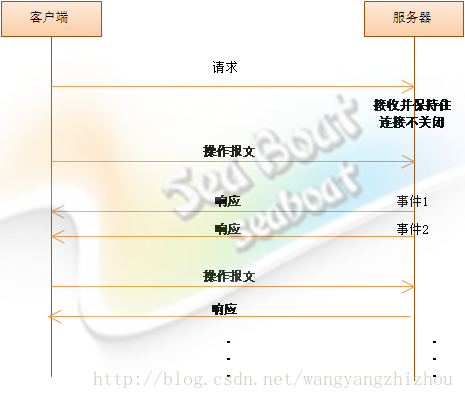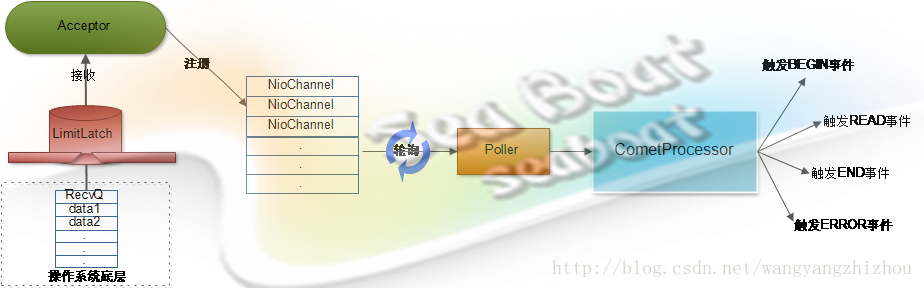Comet模式是一种服务器端推技术，它的核心思想提供一种能让当服务器端往客户端发送数据的方式。Comet模式为什么会出现？刚开始人们在客户端通过不断自动刷新整个页面来更新数据，后来觉得体验不好又使用了AJAX不断从客户端轮询服务器更新数据，然后是使用Comet模式由服务器端通过长连接推数据。Comet模式能大大减少发送到服务器端的请求从而避免了很多开销，而且它还具备更好的实时性。`public` `interface` `CometProcessor ``extends` `Servlet{`
`  ``public` `void` `event(CometEvent event)`
`    ``throws` `IOException, ServletException;`
`}`

①　BEGIN，表示请求开始，此时客户端连接已被接收。

③　END，表示请求结束，此时客户端连接将被断开。

④　ERROR，表示发生了IO异常，一般将会结束此次请求并且连接会被断开。

`public` `class` `CometServlet ``extends` `HttpServlet ``implements` `CometProcessor {`

`  ``protected` `ArrayList connections = ``new` `ArrayList();`

`  ``public` `void` `event(CometEvent event) ``throws` `IOException, ServletException {`
`    ``HttpServletRequest request = event.getHttpServletRequest();`
`    ``HttpServletResponse response = event.getHttpServletResponse();`
`    ``if` `(event.getEventType() == CometEvent.EventType.BEGIN) {`
`      ``synchronized` `(connections) {`
`        ``connections.add(response);`
`      ``}`
`    ``} ``else` `if` `(event.getEventType() == CometEvent.EventType.ERROR) {`
`      ``synchronized` `(connections) {`
`        ``connections.remove(response);`
`      ``}`
`    ``}``else` `if` `(event.getEventType() == CometEvent.EventType.END) {`
`      ``synchronized` `(connections) {`
`        ``connections.remove(response);`
`      ``}`
`    ``} ``else` `if` `(event.getEventType() == CometEvent.EventType.READ) {`
`      ``InputStream is = request.getInputStream();`
`      ``byte``[] buf = ``new` `byte``[``512``];`
`      ``do` `{`
`        ``int` `n = is.read(buf);`
`        ``if` `(n > ``0``) {`
`          ``System.out.println(``new` `String(buf, ``0``, n));`
`        ``} ``else` `if` `(n < ``0``) {`
`          ``return``;`
`        ``}`
`      ``} ``while` `(is.available() > ``0``);`
`    ``}`
`  ``}`
`}`Tomcat